SEARCH HOMEMath Central Quandaries & QueriesQuestion from luis, a student: 3x-2//4-3x=12Hi Luis. I'm interpreting your question as this: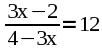I think what you must be having trouble with is sorting out that fraction.

If it was a simpler fraction I think you would find it easier to see what to do. Let's just say it is A over B. You would do this: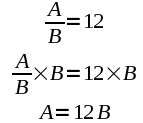Right? Well, it is no different with a question like yours, it is just that the fraction LOOKS harder -- the same steps are actually involved.

For example, here is a question a lot like yours: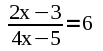To get rid of the fraction, you multiply both sides by the denominator, just like we did in A/B above when we multiplied by B: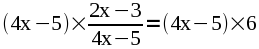Then you can finish the solution in the usual way: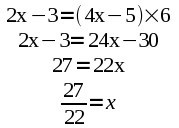Now that you have a close example, follow this model and solve your question.

Hope this helps,
Stephen La Rocque.Math Central is supported by the University of Regina and The Pacific Institute for the Mathematical Sciences.